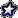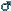###Author Topic: Mono Spaced Word Search Font Test  (Read 6347 times)

0 Members and 1 Guest are viewing this topic.

####Jade Sinapu

• Hero Member
• Species: Wolf / GSD
•• Still hanging in there...
••• Posts: 1578##### Mono Spaced Word Search Font Test
« on: November 19, 2018, 09:31:59 pm »
using Orange size 12 Courier
letters are tab spaced

X   U   M   Y   S   E   B   E   P   J   V   T   N   C   Y
N   S   Z   M   A   L   N   I   P   M   F   G   X   K   E
G   G   J   F   A   W   I   B   M   O   Z   K   U   J   L
T   C   F   C   E   C   Y   Y   P   Y   J   H   Q   E   Q
N   P   K   H   N   J   Q   M   O   B   R   O   W   N   R
X   S   L   O   Z   C   A   B   T   A   S   D   J   A   K
U   W   O   J   X   U   C   L   K   Q   G   W   W   Y   G
J   U   O   T   X   S   D   U   H   S   U   Z   E   Y   M
W   E   L   P   R   U   P   E   B   R   R   L   K   D   D
I   E   Y   U   Z   K   S   G   F   V   L   M   W   Z   T
Z   G   T   D   S   P   L   L   R   O   R   S   L   I   W
J   N   T   D   E   T   I   H   W   E   P   I   N   K   Q
F   A   E   A   C   H   S   F   H   D   E   R   E   U   A
M   R   P   Q   V   H   Z   M   F   D   F   N   F   I   Z
F   O   I   F   O   J   Y   J   U   J   O   S   O   S   R

BLACK
GREEN
PINK
BROWN
YELLOW
BLUE
PURPLE
ORANGE
RED
WHITE
Bear your soul and take control
If the wolves are howling outside your door
Invite them in and make them beg for more!
(Name that tune!)